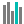#F.DIST (PQL - xl)

This function calculates the F probability distribution, to see degrees of diversity between two sets of data

• Library: PQL \ Spreadsheet \ Statistical
• Compatibility: Any content (regardless of data source) in the Tabulate spreadsheet module

#### Syntax

F.DIST(x, deg freedom1, deg freedom2, cumulative)

##### Function Arguments
 Name Description Type Optional x Value at which to evaluate the function Number deg freedom1 Number of degrees of freedom for the numerator; if not an integer, the number is truncated Number deg freedom2 Number of degrees of freedom for the denominator; if not an integer, the number is truncated Number cumulative A logical value that determines the form of the function. If cumulative is TRUE, this function returns the cumulative distribution function; if FALSE, it returns the probability density function StringOrNumber